Examples

Chapter 6 Class 12 Application of Derivatives
Serial order wise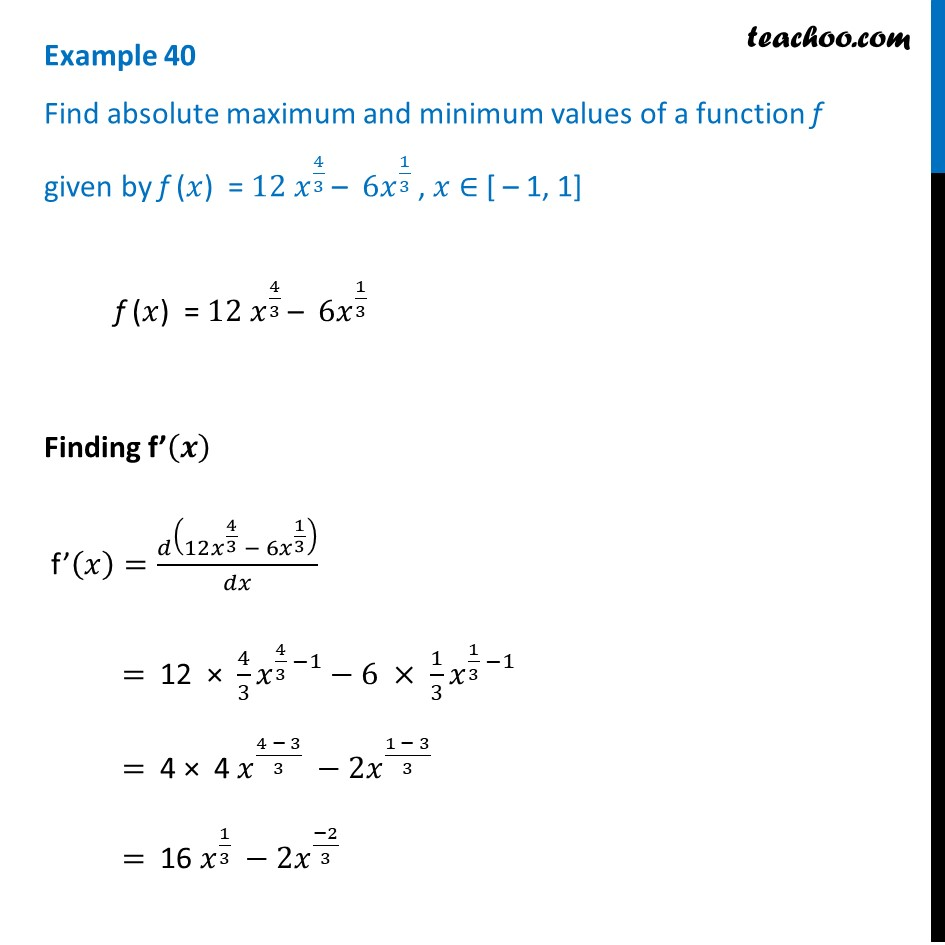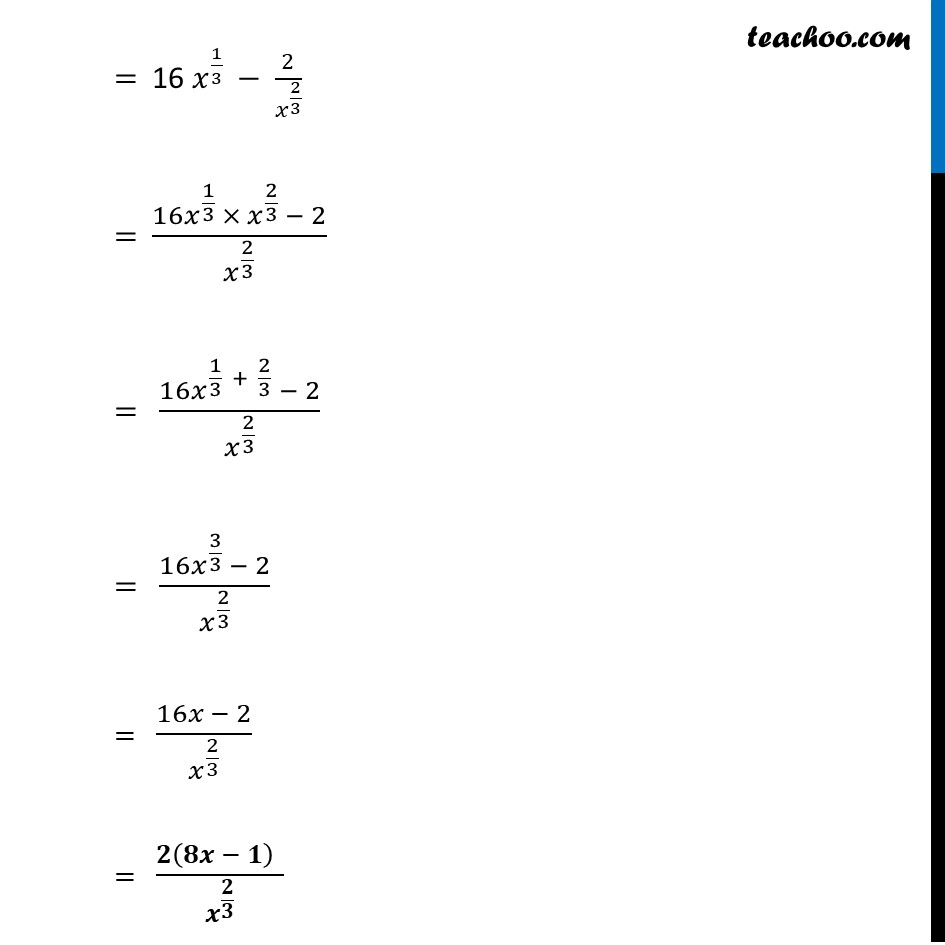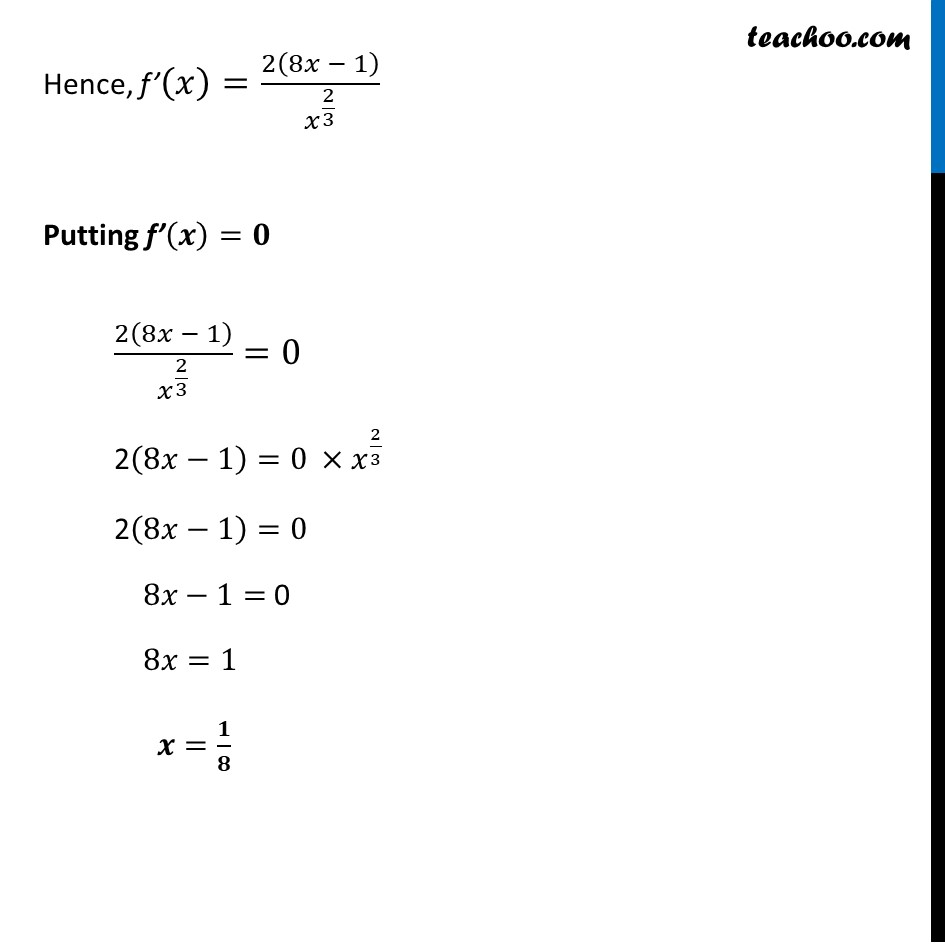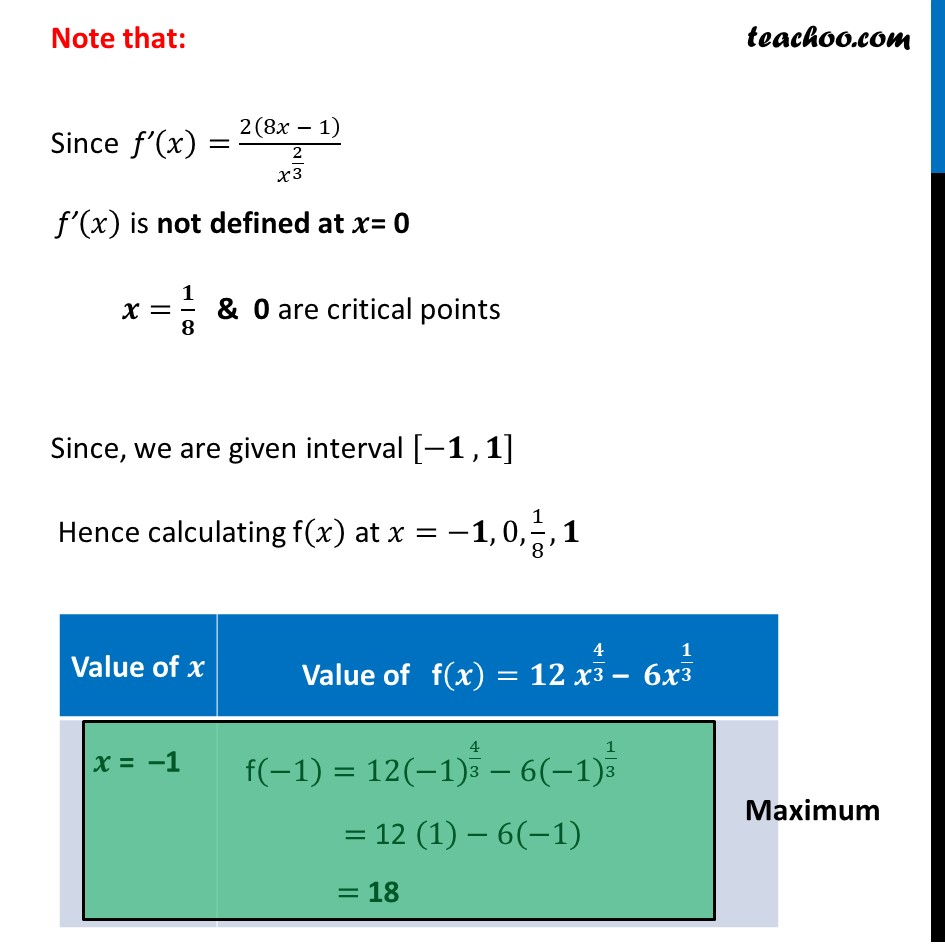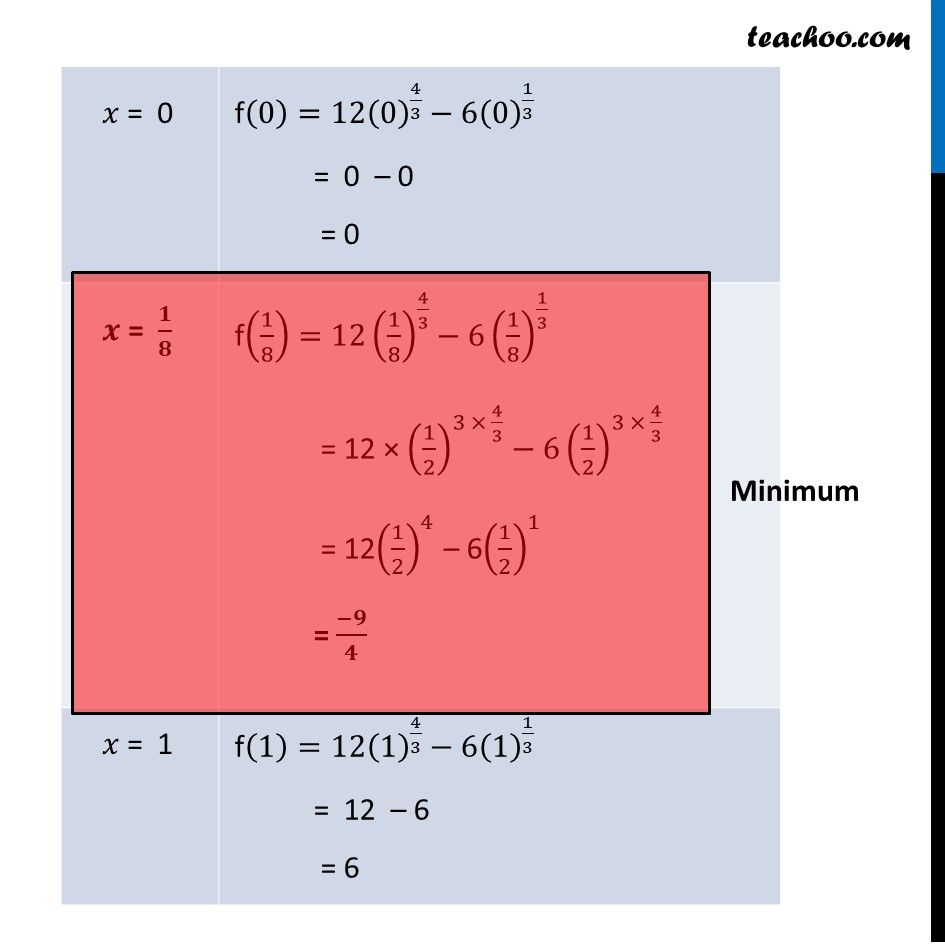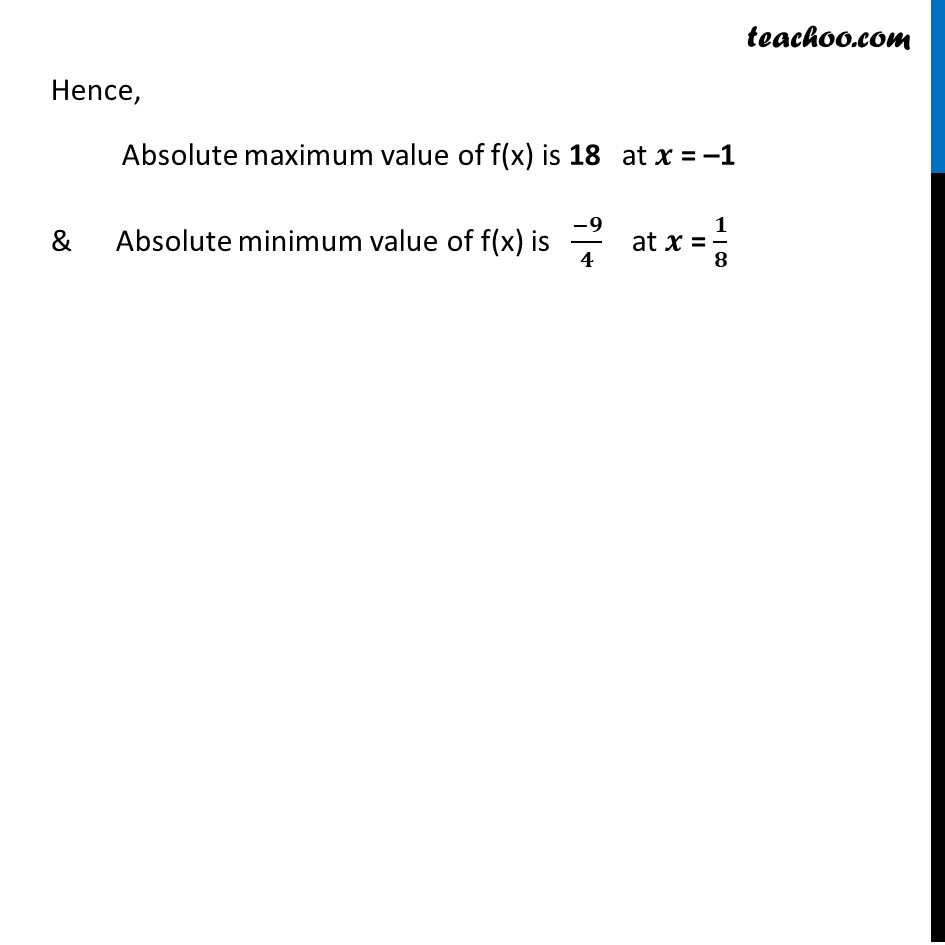Learn in your speed, with individual attention - Teachoo Maths 1-on-1 Class

### Transcript

Example 28 Find absolute maximum and minimum values of a function f given by f (𝑥) = 〖12 𝑥〗^(4/3) – 〖 6𝑥〗^(1/3) , 𝑥 ∈ [ – 1, 1] f (𝑥) = 〖12 𝑥〗^(4/3) – 〖 6𝑥〗^(1/3) Finding f’(𝒙) f’(𝑥)=𝑑(12𝑥^(4/3) − 6𝑥^(1/3) )/𝑑𝑥 = 12 × 4/3 𝑥^(4/3 −1)−6 × 1/3 𝑥^(1/3 −1) = 4 × 4 𝑥^((4 − 3)/3) −2𝑥^((1 − 3)/3) = 16 𝑥^(1/3) −2𝑥^((−2)/3) = 16 𝑥^(1/3) − 2/𝑥^(2/3) = (16𝑥^(1/3) × 𝑥^(2/3) − 2)/𝑥^(2/3) = (16𝑥^(1/3 + 2/3) − 2)/𝑥^(2/3) = (16𝑥^(3/3) − 2)/𝑥^(2/3) = (16𝑥 − 2)/𝑥^(2/3) = 𝟐(𝟖𝒙 − 𝟏)" " /𝒙^(𝟐/𝟑) Hence, f’(𝑥)=2(8𝑥 − 1)/𝑥^(2/3) Putting f’(𝒙)=𝟎 2(8𝑥 − 1)/𝑥^(2/3) =0 2(8𝑥−1)=0 ×𝑥^(2/3) 2(8𝑥−1)=0 8𝑥−1= 0 8𝑥=1 𝒙=𝟏/𝟖 Note that: Since f’(𝑥)=2(8𝑥 − 1)/𝑥^(2/3) f’(𝑥) is not defined at 𝒙= 0 𝒙=𝟏/𝟖 & 0 are critical points Since, we are given interval [−𝟏 , 𝟏] Hence calculating f(𝑥) at 𝑥=−𝟏, 0, 1/8, 𝟏 f(0)=12(0)^(4/3)−6(0)^(1/3) = 0 – 0 = 0 f(1)=12(1)^(4/3)−6(1)^(1/3) = 12 – 6 = 6 Hence, Absolute maximum value of f(x) is 18 at 𝒙 = –1 & Absolute minimum value of f(x) is (−𝟗)/𝟒 at 𝒙 = 𝟏/𝟖